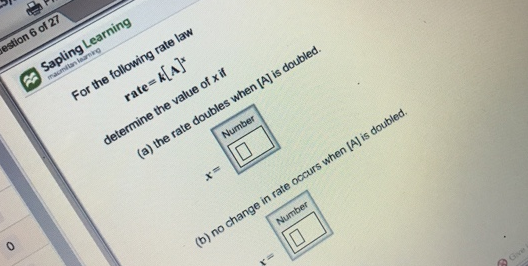# Problem: For the following of rate lawrate = k[A]xdetermine the value of x if (a) the rate double when [A] is doubled.(b) no change in rate occurs when [A] is doubled.

###### FREE Expert Solution
92% (192 ratings)###### Problem Details

For the following of rate law

rate = k[A]x

determine the value of x if

(a) the rate double when [A] is doubled.

(b) no change in rate occurs when [A] is doubled.